The Geometry of Linear Systems

Linear system as a graph

Given the linear system
 5x - 2y = 16 3x + 4y = 20
We can draw each equation as a line on a graph: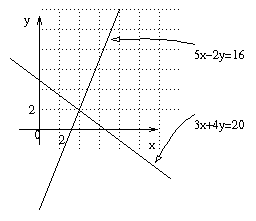One line goes though all the points (x,y) where 5x-2y=16, and the other line goes through all the points (x,y) where the 3x+4y=20. Where the two lines meet, we get a point that satisfies both equations, and we can see right away that this is (x,y)=(4,2).
Aside: Notes on drawing graphs.
Here are a few points to remember: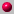Label the axes (eg, x,y, here)Indicate scale (eg, the 0,2)Label graph, eg the "5x-2y=16"Slope and y intercept define a lineMake sure the graph is clear. Not too cluttered.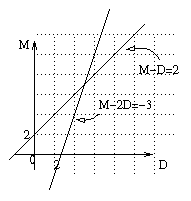The donkey mule example:
We saw this in lecture one:
 M - 2D = -3 M - D = 2

Now we can draw the graph, on the right, and from the graph see that the solution is (D,M)=(5,7).
But sometimes a graph is not as accurate as algebra. Do the lines _really_ intersect at (5,7)? Maybe it's just very close, eg, (5.23,7.05)? Well, we know, because we've done the algebra, that the answer really is (5,7).
A graph needs algebra to back it up. But algebra needs the geometry to give us and understanding of what is going on. We can use our geometric intuition to understand the algebra.

Different kinds of solutions to Linear Equations

How can two lines meet?

How many solutions will a linear system have? We only got one possibility for the Mule and Donkey problem. Why not more? From the graph we see why - two lines can only intersect in one point. Unless they intersect in no points, or infinitely many points.
Different ways lines can meet: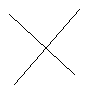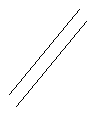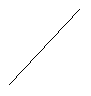One point no points infinitely many points general case parallel lines equal lines

Example 1: infinitely many solutions

Shop W&Z sells a pack of one piece of cheese and 2 crackers for 1\$, or 2 pieces of cheese and 4 crackers for 2\$. How much does cheese cost? How much do crackers cost?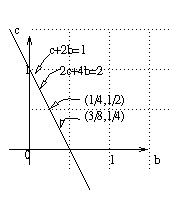We assume that this means:
 c + 2b = 1 2c + 4b = 2

Where c is the price of cheese and b is the price of crackers. (These are biscuits in England, but I can't spell that anyway.)
But when we draw the lines, we find that both equations give the same line. We can't find out the price, it might be (b,c)=(1/4,1/2); it could be (b,c)=(3/8,1/4); it's not very likely to be (b,c)=(0,1).
There are infinitely many solutions. All we can say is c=1-2b. (and something about c and b being non negative.)

Example 2: An inconsistent example: parallel lines

How about shop U&V? It sells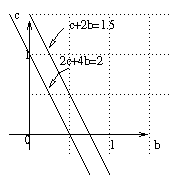• 1 piece of cheese and 2 crackers = 1.50\$
• or 2 pieces of cheese and 4 crackers = 2.00\$

What can we deduce about the costs of cheese and crackers?
What does the graph look like?
The lines are parallel. So there are no solutions.
The system is inconsistent.
So this means that there was something wrong about our assumption that cheese and crackers had the same value in both packs. We can save money by buying more. This would be "inconsistent" if you only ever paid for exactly what you got.

Row reduction for an inconsistent system

To tell geometrically if there is no solution, and that the system is inconsistent, you just see if the lines are parallel.
To tell algebraically by doing row reduction, you look at your reduced matrix: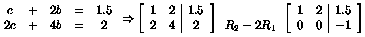And that second line, with the zeros except for the -1 at the end, tells you something must be wrong, since that line means
0c+0b=-1
which is impossible.
Generally if there is a reduced matrix with a non zero entry at the end of a row of zeros, the system is inconsistent.

In the case where there was only one line, we didn't have enough information. Sometimes we have more information than we need. (Later we'll come back to this idea, and see that the set of equations is not linearly independent.) Extra data might be a useful check, but if we assume consistency, we don't really need it.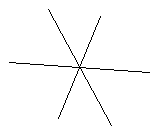Exercise: Finish off the example by finding a question to fit it.

Example 4: Another way of being inconsistent

You might not have parallel lines but still be inconsistent.
How? What happens in the following example? Example: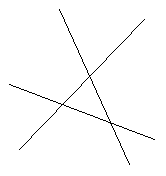Exercise: Finish of the example by making up a problem to fit it.

More Variables---More Dimensions

With 3 variables, the equations describe planes in 3 dimensional space. Usually, 3 equations give 3 planes, which intersect in one point. But sometimes we get inconsistent systems, or systems with infinitely many solutions.
With n variables, if we could draw in n dimensions, we'd expect to need n "hyper-planes" to get a unique solution. And things would be consistent sometimes, or inconsistent, or there would be infinitely many solutions.
We can't really draw n dimensions for n>3, but we can still use some geometric intuition gained from 1,2,3 dimensions.
There are some pictures on page (?) of the text book which give some different possibilities.

Geometric meaning of row reduction

What does row reduction mean? We start off with one set of equations, and we get to another set, which have the same solutions. This is like taking a different set of lines that go through the same point. But if the lines are parallel to the axis, it's much easier to read off the solution.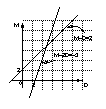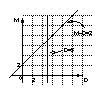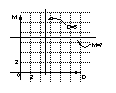Given Equations Echelon form Reduced Echelon

The Mule could have said a lot of different things to the Donkey; in fact, for each line through the point (D,M)=(6,4) he could choose a different fact about their loads. Just pick any two lines in the picture below, and make up a riddle the mule asked the donkey.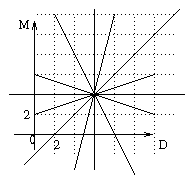Vectors

Text book section 2.1

Basically, you can think of a vector space of dimension n as a collection of lists of n numbers.
The numbers are real (ie., all the numbers you come across in everyday life), and there are n of them. So, we call the set of all strings of n real numbers a real vector space of dimension n and use the following symbol to refer to it: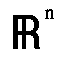(I shall write R^n, since I can type that more easily.)
Eg: R^4 consists of all things that look like (a,b,c,d), where a,b,c,d are real numbers.
Eg, (1,2,3,4),(2,7,0.5^(1/2),-1) are in R^4.

What does a vector mean?

A vector is just a string of numbers, but a string of numbers can be interpreted in all kinds of different ways.
• It can represent the amounts of various quantities
• It can represent a point on a graph
• It can represent a magnitude in a certain direction
For Example
• Amounts of eggs, milk etc (in examples for recipes below)
• co-ordinates of a point on a map of Kingston
• Applied math and physics problems.

What can you do with vectors?

Eg: The omelet will use the following ingredients:
(eggs,sugar,milk,butter,chocolate,onions,fish paste)=(6,0,0,1,0,3,4)
(units are in cups)
The cake will use
(eggs,sugar,milk,butter,chocolate,onions,fish paste)=(1,2,2,1,10,0,0)
So the whole meal needs:
(eggs,sugar,milk,butter,chocolate,onions,fish paste)=(6,0,0,1,0,3,4)+(1,2,2,1,10,0,0) =(7,2,2,2,1,10,3,4)

Multiply by a scaler

Scalar is just a fancy word for a "number", calling it a scalar stresses that it's not a vector. (And a scaler is something that climbs up walls, maybe.)
Eg: For one cake, I need to buy
(eggs,sugar,milk,butter,chocolate,onions,fish paste)=(1,2,2,1,10,0,0)

So when I make three cakes, I need to buy
(eggs,sugar,milk,butter,chocolate,onions,fish paste)=3(1,2,2,1,10,0,0)=(3,6,6,3,30,0,0)

Where do vectors come from?

Vectors come up in any problem where the solutions (or possible solutions) can be combined in the above ways. There are a lot of problems where if you have two solutions, you can add them together to get a third. This is very useful, since it means that if you want to know _all_ possible solutions, you just have to know "enough" "basic" solutions, and that will tell you everything. This is a really important idea in mathematics. We will talk about what "enough" means, and what "basic" means later. ("Enough" and "basic" are not technical words; the corresponding technical words are spanning and basis.)
Eg. Problem: What can I include in my calorie free diet?

Answer 2: a stick of celery!

Then from these two solutions, we get infinitely many more, eg:

Answer 3: two carrots and 5 sticks of celery!
Answer 4: half a carrot and a quarter of a stick of celery.

We represent these solutions as 1: (1,0), 2: (0,1), 3: (2,5), 4: (1/2,1/4)
So carrots and celery are more "basic" solutions than answers 3 and 4; but we clearly have not got "enough" basic solutions to make a very interesting diet.

Geometrical interpretation

We can interpret (a,b) as a point in R^2, or a vector telling us how to get to a point in R^2 from (0,0):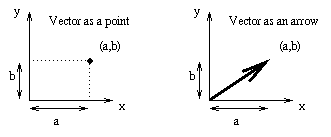Geometrically, vector addition means joining the corresponding arrows end to end: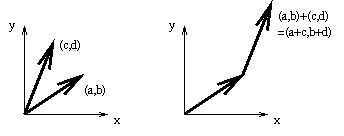It doesn't matter which order you add them in.

Multiplication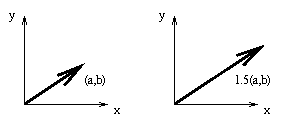Cute application of the fact that whichever way you add up a collection of vectors, you get the same answer.

Here's the problem. You have a lot of circles, all of radius one. You arrange them in a pyramid shape, so that the bottom row has n circles, all touching a straight line; the next row has n-1 circles, each one touching two circles on the row below, and so on, each row having one less circle, until at the top there is only one circle: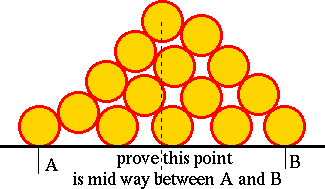Prove that the center of the top circle lies directly above the mid point of the centers of the outer circles of the bottom row.
There is a very quick geometrical proof of this using the fact that whatever way you add some vectors, you get the same answer. The proof is easy, but it might be tricky to spot. You can email me if you get stuck for too long. I learned this problem from a graduate student at Queens, Adam Brown.

The Span of a set of vectors

We started off with lines. A linear equation can be represented by a line.
Example: All the points (x,y) satisfying
5x-2y=16
lie on a straight line: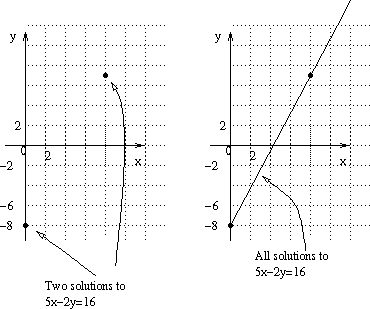Probably you learned at school how to draw a line by finding the y intercept and the slope. The line is given by
y=cx+b,
and c tells you the slope, and b tells you the y intercept.
So for 5x-2y=16, we can rewrite this as
y=2.5x-8

So from this we can see that the slope is 2.5, and the y intercept is 8.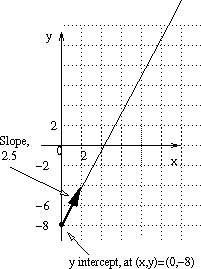Our new way of thinking is in terms of vectors.
Insead of saying "the y-intercept is -8", we say "the line goes through the point (0,-8)".
The slope is 2.5 means that when x changes by 1, y changes by 2.5. The change is (1,2.5). If x changes by 2, y changes by 5, so the change is (2,5)=2(1,2.5). The change in moving from one point on the line to another on the line will always be a multiple of (1,2.5). So the vector (1,2.5) is another way of saying the slope is 2.5.
Any point on the line is given by (0,-8) + t(1,2.5) for some number t.
The vector way of thinking is summarized below:
Old way of describing New way of describing
The y intercept is b The line passes through the point (0,b)
The slope of the line is c The line goes in the direction (1,c)
Equation is y=cx+b The vector equation is (0,b)+t(1,c)

This new notation is useful. It reminds us that you start from (0,-8), and go in the direction (1,2.5); but it's more versitile, since we could start at another point on the line, eg, (2,-3), and go in the direction (1,2.5), and still get everywhere on the line. So we can also write the vector equation as
(2,-3)+t(1,2.5)

The line y=bx

We have two things happening. One is that there is a y intercept. The other is this direction vector.
To make things simpler, we just look at one thing at a time. Later we'll think about the intercept. For now we'll let the intercept be zero.
Then the line is given by
y=bx
And to get to any point on the line, you just need to take some multiple of the point (1,b).
The line is all the points you can get to using the vector (1,b). This is called the span of the vector (1,b).
Eg, Take the equation y=2x (which is the same as the points with y-2x=0):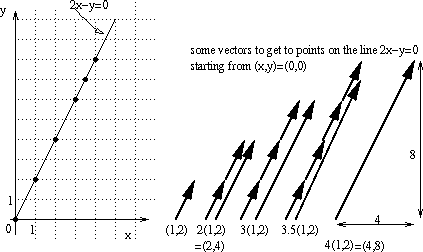Then (1,2) is a point on this line. So is (2,4), and (2,4)=2(1,2). Other points:
 3(1,2) = (3,6) 4(1,2) = (4,8) 5.5(1,2) = (5.5,11) 1.3(1,2) = (1.3,2.6)

These are some of the points in the span of (1,2). The span includes all the points you can get to.
Next, what about a plane, if we are in a higher dimension?
To simplify, we again assume that the plane goes through the origin, at (0,0,0). What defines the plane? How can you specify all the points you can get to?
What happens is that we can take just two directions, and use them to get everywhere. This is called their span.
Back at the calorie problem; the span of the vector (1,0) of one carrot and no celery, and (0,1), no carrot, and 1 stick of celery, is any thing of the form a(1,0)+b(0,1), which means a carrots, and b sticks of celery, (a,b).

Span and linear systems

Span is very relevent to linear systems, since finding is something is in the span of some vectors is the same problem as solving a linear system.

Vector equations

(I need to add a couple more graphs to this section, I'll do that soon)

We've already seen two ways of presenting the information given by a linear system. Remember the Mule and Donkey problem from lecture 1? This was given by a linear system:
 M - 2D = -3 M - D = 2

The next way of writing it is as an augemented matrix.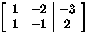Now we'll see a third way of looking at the information: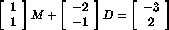This is a vector equation.
We've already solved the linear system by solving the augmented matrix. We know that
7(1,1)+5(-2,-1)=(-3,2),

(In more detail, this is because 7(1,1)=(7,7), and 5(-2,-1)=(-10,-5), so
7(1,1)+5(-2,-1)=(7,7)+(-10,-5)=(7-10,7-5)=(-3,2))
Geometrically, this describes how to use the vectors (1,1) and (-2,-1) to get to the point (-3,2):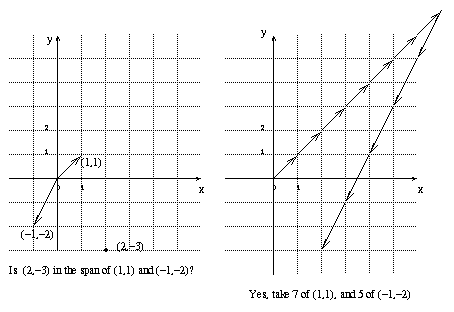We say (-3,2) is in the span of (1,1) and (-2,-1).
The span of (1,1) and (-2,-1) is all the points you can get to using these two vectors. In this case, you can get to any point in R^2 (remember, R2 means pairs of two real numbers, so that means any point on the graph.)
Now we can look at some of the other examples from the point of view of vector equations.

Example 2 (inconsistant case)

For the system above,
 c + 2b = 1.5 2c + 4b = 2

The vector equation is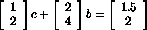We found that there was no solution to this linear system. In the new notation, that is because the vectors were in the same direction, so they can only get to points which are multiples of (1,2), so (1.5,2) can not be reached.
We say that (1.5,2) is not in the span of (1,2) and (2,4).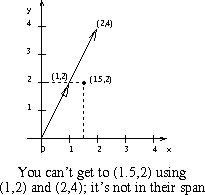Example 3 (infinitely many solutions)

Now look at the other example with linear system:
 c + 2b = 1 2c + 4b = 2

The vector equation (as row vectors, rather than column vectors), is
(1,2)c+(2,4)b=(1,2)

now there are infinitely many ways we can use (1,2) and (2,4) to get to (1,2).
Eg, with (c,b)=(1/2,1/4), we have (1,2)*(1/2)+(2,4)*(1/4)=(1/2,1)+(1/2,1)=(1,2).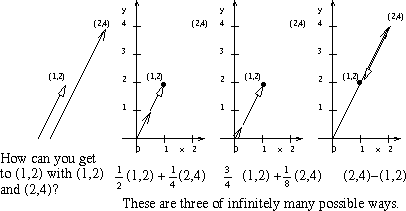Example 4 (Three dimensional case)

We can write
 (1,0,0) to mean "go one unit North", (0,1,0) to mean "go one unit East", (0,0,1) to mean "go one unit up".

Can we get to the point (0,0,1) using (1,0,0) and (0,1,0)?
No, it's not in their span; we can never go up, no matter how far we go North or East, South or West (these are North and East in the negative direction).
Trying to find a way to get to (0,0,1) with (1,0,0) and (0,1,0) is like trying to solve the vector equation:
 / 1 \ / 0 \ / 0 \ | 0 | x + | 1 | y = | 0 | \ 0 / \ 0 / \ 1 /

Which is the same as solving the augmented matrix:
 / 1 0 | 0 \ | 0 1 | 0 | \ 0 0 | 1 /

But this is inconsistant, because there is a row of zeros that does not end in a zero.